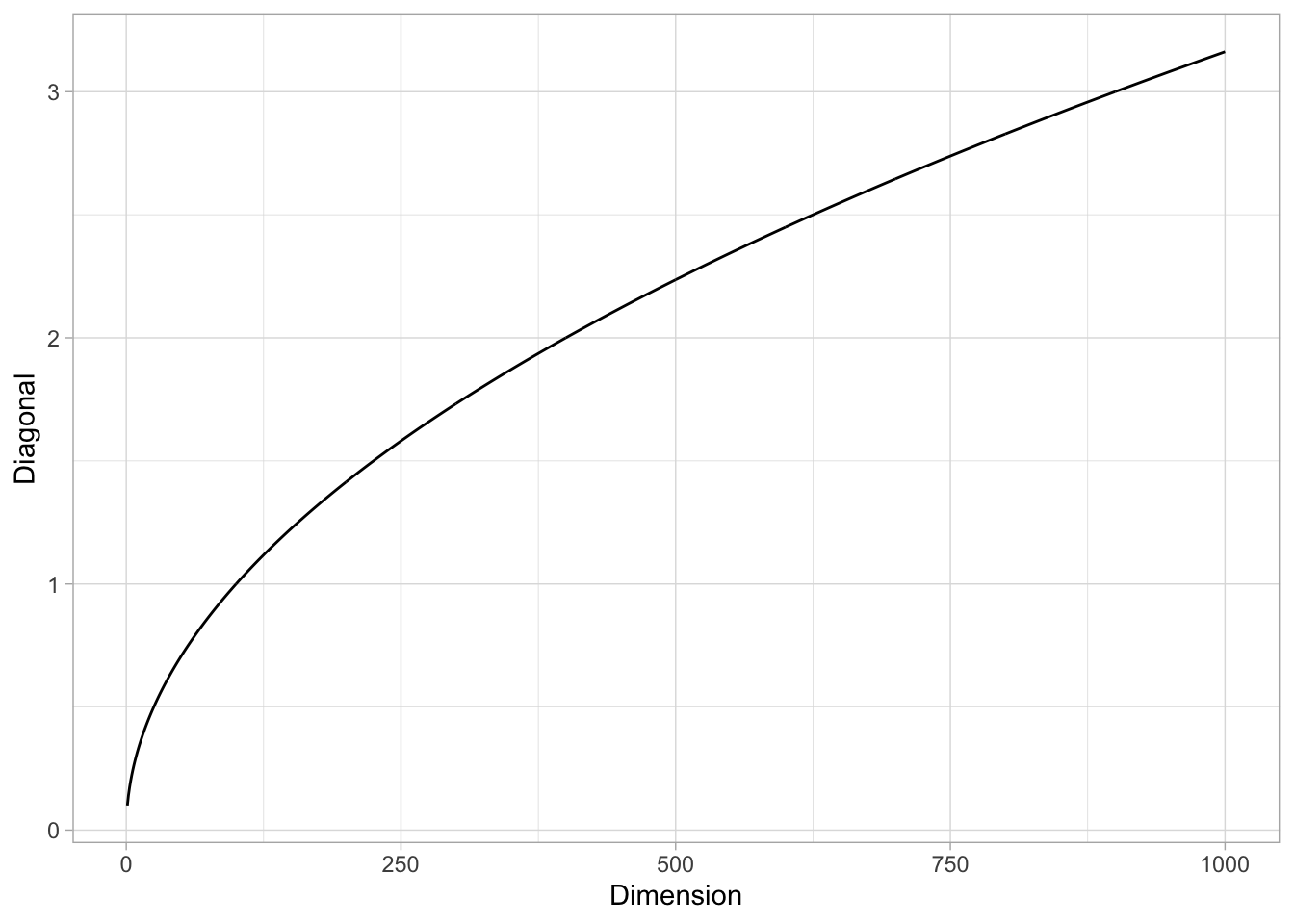# Exploring the Curse of Dimensionality - Part I.

In this post I want to present the notion of curse of dimensionality following a suggested exercise (Chapter 4 - Ex. 4) of the book An Introduction to Statistical Learning, written by Gareth James, Daniela Witten, Trevor Hastie and Robert Tibshirani.

When the number of features $$p$$ is large, there tends to be a deterioration in the performance of KNN and other local approaches that perform prediction using only observations that are near the test observation for which a prediction must be made. This phenomenon is known as the curse of dimensionality, and it ties into the fact that non-parametric approaches often perform poorly when $$p$$ is large. We will now investigate this curse.

1. Suppose that we have a set of observations, each with measurements on $$p = 1$$ feature, $$X$$. We assume that $$X$$ is uniformly (evenly) distributed on $$[0,1]$$. Associated with each observation is a response value. Suppose that we wish to predict a test observation’s response using only observations that are within $$10$$% of the range of $$X$$ closest to that test observation. For instance, in order to predict the response for a test observation with $$X = 0.6$$ we will use observations in the range $$[0.55,0.65]$$. On average, what fraction of the available observations will we use to make the prediction?

Let us prepare the notebook.

library(glue)
library(tidyverse)

Let $$\lambda:= 0.1$$ represent the locality input parameter. i.e $$10$$%.

lambda <- 0.1

Let us generate $$X$$, i.e. the sample observations.

# Set dimension.
p <- 1

# Number of sample points.
n0 = 100

# Generate observation matrix
X <- runif(n = n0, min = 0, max = 1)  %>% matrix(ncol = p)

Given $$z_0 \in [0,1]$$, let us define a function which returns the $$\lambda$$-interval. We need to consider separate cases, depending wether the $$z_0$$ has distance less than $$\lambda/2$$ to some of the boundary points.

GenerateInterval <- function (z0, lambda) {
#'Generate lambda-interval
#'
#'@param z0 point in the unit interval.
#'@param lambda locality parameter.
#'@return lambda-interval of z0.

if (z0 < lambda/2) {

u.min <- 0
u.max <- lambda

} else if (z0 < 1 - lambda/2) {

u.min <- z0 - lambda/2
u.max <- z0 + lambda/2

} else {

u.min <- 1 - lambda
u.max <- 1

}

interval <- c(u.min, u.max)

return(interval)
}

Let us consider some examples:

z0 <- 0.6

GenerateInterval(z0 = z0, lambda = lambda)
##  0.55 0.65
z0 <- 0

GenerateInterval(z0 = z0, lambda = lambda)
##  0.0 0.1

Now let us write a function which verifies if a point $$x \in [0,1]$$ belongs to a given interval.

IsInInterval <- function (x, interval) {
#'Evaluate wether a given point belongs to the given interval.
#'
#'@param x point in the unit interval.
#'@param interval vector of length two indicating interval limits.
#'@return boolean indicating wether x belongs to the given interval.

(( x > interval ) & ( x < interval ))
}

For example,

z0 <- 0.6

interval <- GenerateInterval(z0 = z0, lambda = lambda)
IsInInterval(x = 0.5, interval = interval)
##  FALSE
IsInInterval(x = 0.57, interval = interval)
##  TRUE

Next, given any point and an observation matrix $$X$$ we want to count the number of points in the sample which belong to the $$\lambda$$-interval of the point.

CountAvailableObservations <- function (z0, X, lambda) {
#' Count observations in the lambda-interval of a point.
#'
#'@param z0 point in the unit interval.
#'@param X observation matrix.
#'@return number of samples in the lambda-interval of z0.

interval <- GenerateInterval(z0 = z0, lambda = lambda)

condition <- IsInInterval(x = X, interval = interval)

count.obs <-  X[condition] %>% length

return(count.obs)
}

Now we write a simulation function.

RunSimulation1 <- function(lambda, n0, n1, N) {
#' Run simulation.
#'
#'@param lambda locality parameter.
#'@param z0 point in the unit interval.
#'@param n0 number of samples in the observation matrix.
#'@param n1 number of samples in the prediction matrix.
#'@param N number of times to run the count.
#'@return vector of fraction counts.

# Run over simulation iterations.
1:N %>% map_dbl(.f = function(k) {
# Generate random observation samples.
X <- runif(n = n0, min = 0, max = 1)
# Generate prediction grid.
sample.grid <- runif(n = n1, min = 0, max = 1)

sample.grid %>%
map_dbl(.f = ~ CountAvailableObservations(z0 = .x, X= X, lambda = lambda)) %>%
mean

}) / n1
}

Let us plot the distribution.

sim.1 <- RunSimulation1(lambda = 0.1,
n0 = 100,
n1 = 100,
N = 100)

mean.sim.1 <- mean(sim.1)

sim.1 %>%
as_tibble() %>%
ggplot() +
theme_light() +
geom_histogram(mapping = aes(x = value),
fill = 'blue',
alpha = 0.7,
bins = 20) +
geom_vline(xintercept = mean.sim.1) +
ggtitle(label = 'Fraction Simulation Distribution for p = 1')
## Warning: Calling as_tibble() on a vector is discouraged, because the behavior is likely to change in the future. Use tibble::enframe(name = NULL) instead.
## This warning is displayed once per session.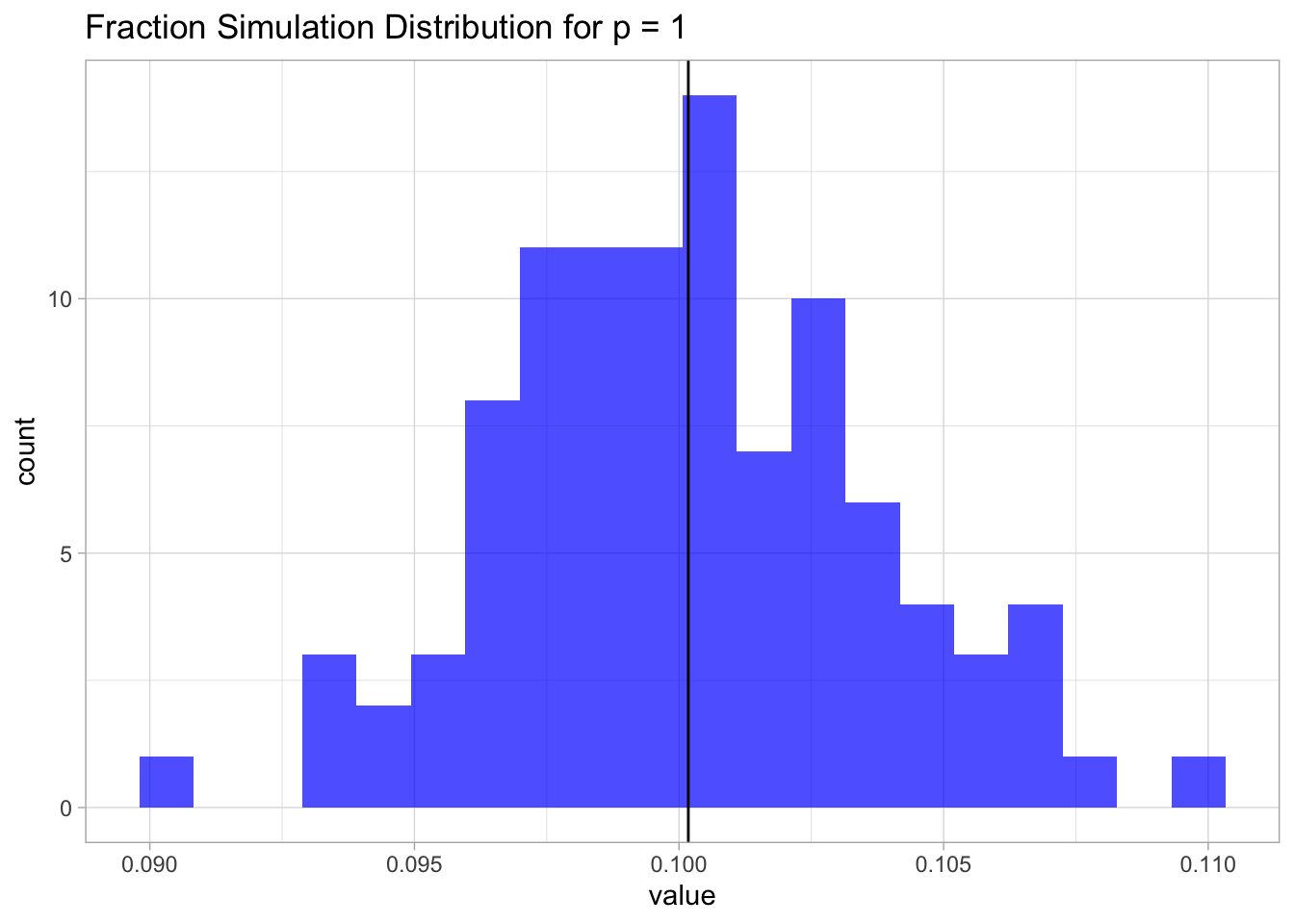This result is not surprising. Since the samples are uniformly distributed on $$[0,1]$$, the fraction is going to be, on average, equal to the length of an interval of length $$\lambda$$, which in this case is just $$0.1$$.

sim.1 %>%
as_tibble() %>%
ggplot() +
theme_light() +
geom_histogram(mapping = aes(x = value),
fill = 'blue',
alpha = 0.7,
bins = 20) +
geom_vline(xintercept = mean.sim.1) +
geom_vline(xintercept = lambda, color = 'red') +
ggtitle(label = 'Fraction Simulation Distribution for p = 1')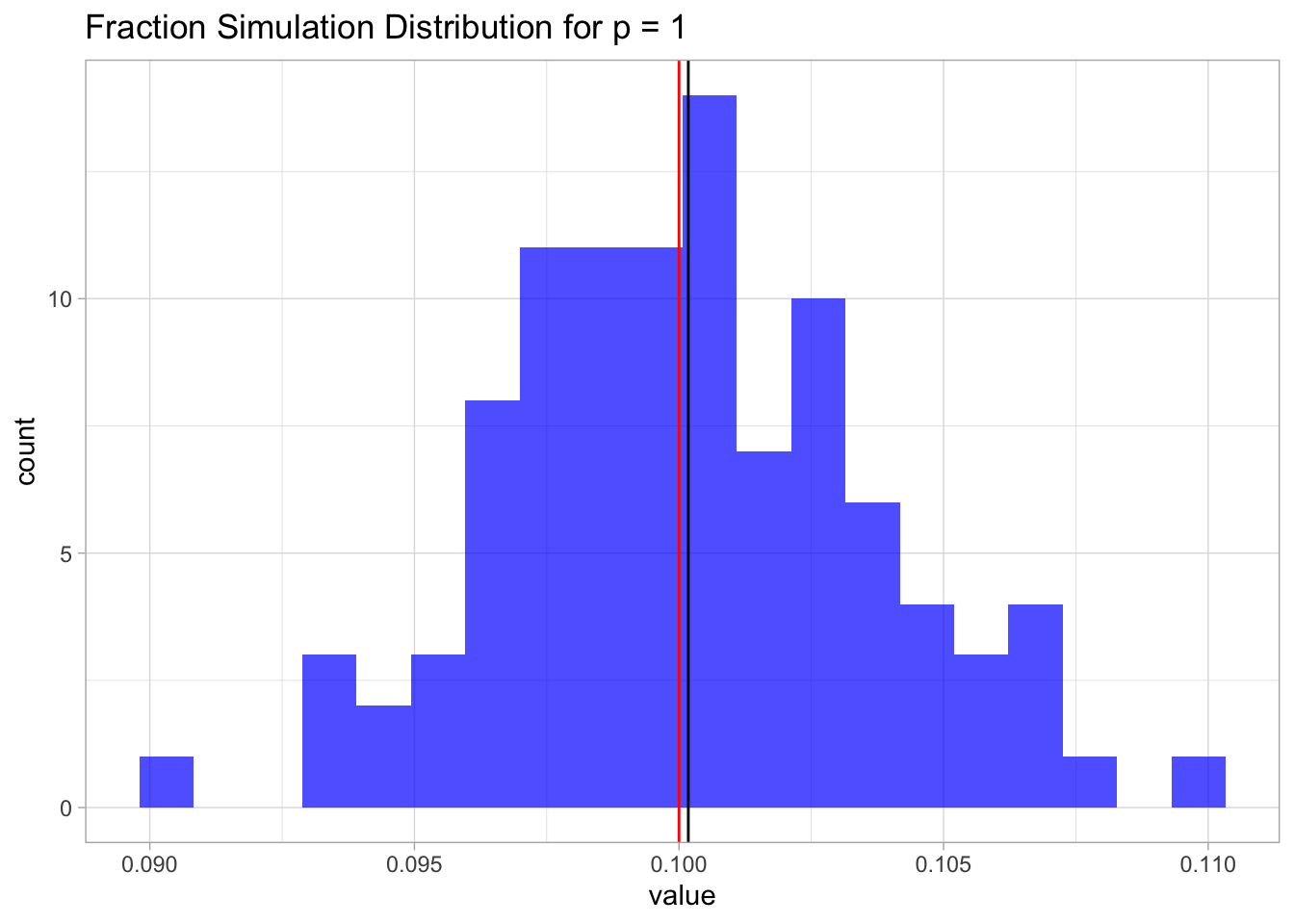1. Now suppose that we have a set of observations, each with measurements on $$p = 2$$ features, $$X_1$$ and $$X_2$$. We assume that $$(X_1,X_2)$$ are uniformly distributed on $$[0,1]\times [0,1]$$. We wish to predict a test observation’s response using only observations that are within $$10$$% of the range of $$X_1$$ and within $$10$$% of the range of $$X_2$$ closest to that test observation. For instance, in order to predict the response for a test observation with $$X_1 = 0.6$$ and $$X2 = 0.35$$, we will use observations in the range $$[0.55, 0.65]$$ for $$X_1$$ and in the range $$[0.3, 0.4]$$ for $$X_2$$. On average, what fraction of the available observations will we use to make the prediction?

We argue similarly as above. We write a simulation function for all $$p>0$$.

RunSimulationP <- function(lambda, p, n0, n1, N) {
#' Run simulation.
#'
#'@param lambda locality parameter.
#'@param z0 point in the unit interval.
#'@param n0 number of samples in the observation matrix.
#'@param n1 number of samples in the prediction matrix.
#'@param N number of times to run the count.
#'@return vector of fraction counts.

# Run over simulation iterations.
1:N %>% map_dbl(.f = function(k) {
# Generate random observation samples.
X <- runif(n = p*n0, min = 0, max = 1) %>% matrix(ncol = p)
# Generate prediction grid.
sample.grid <- runif(n = p*n1, min = 0, max = 1) %>% matrix(ncol = p)

fraction.vect <- sapply(X = 1:n0, FUN = function(i) {

condition.matrix <- sapply(X = 1:p, FUN = function(j) {
# Select a point on the grid.
z0 <- sample.grid[i, j]
# Compute its interval.
interval<- GenerateInterval(z0 = z0, lambda = lambda)
# Check which values in the grid belong to the lambda-interval.
IsInInterval(x = X[, j], interval = interval)
})
# Define condition matrix for each dimension.
condition.vect <- condition.matrix %>%
as_tibble %>%
mutate_all(.funs = as.numeric) %>%
rowSums
# Select points which belong to the lambda-hypercube
# and then compute the fraction.
(condition.vect[condition.vect == p] %>% length) / n0
})

return(mean(fraction.vect))
})
}

Let us run the simulation.

p <- 2

sim.2 <- RunSimulationP(lambda = lambda,
p = p,
n0 = 100,
n1 = 100,
N = 100)
## Warning: as_tibble.matrix() requires a matrix with column names or a .name_repair argument. Using compatibility .name_repair.
## This warning is displayed once per session.
mean.sim.2 <- mean(sim.2)

mean.sim.2 
##  0.010059

Again, since the samples are uniformly distributed on $$[0,1]\times [0,1]$$, this fraction is going to be, on average, equal to the are of a square of length $$\lambda$$, which in this case is just $$0.1^2 = 0.01$$.

Let us plot the distribution.

sim.2%>%
as_tibble() %>%
ggplot() +
theme_light() +
geom_histogram(mapping = aes(x = value),
fill = 'blue',
alpha = 0.7,
bins = 20) +
geom_vline(xintercept = mean.sim.2) +
geom_vline(xintercept = lambda^p, color = 'red') +
ggtitle(label = glue('Fraction Simulation Distribution for p = {p}'))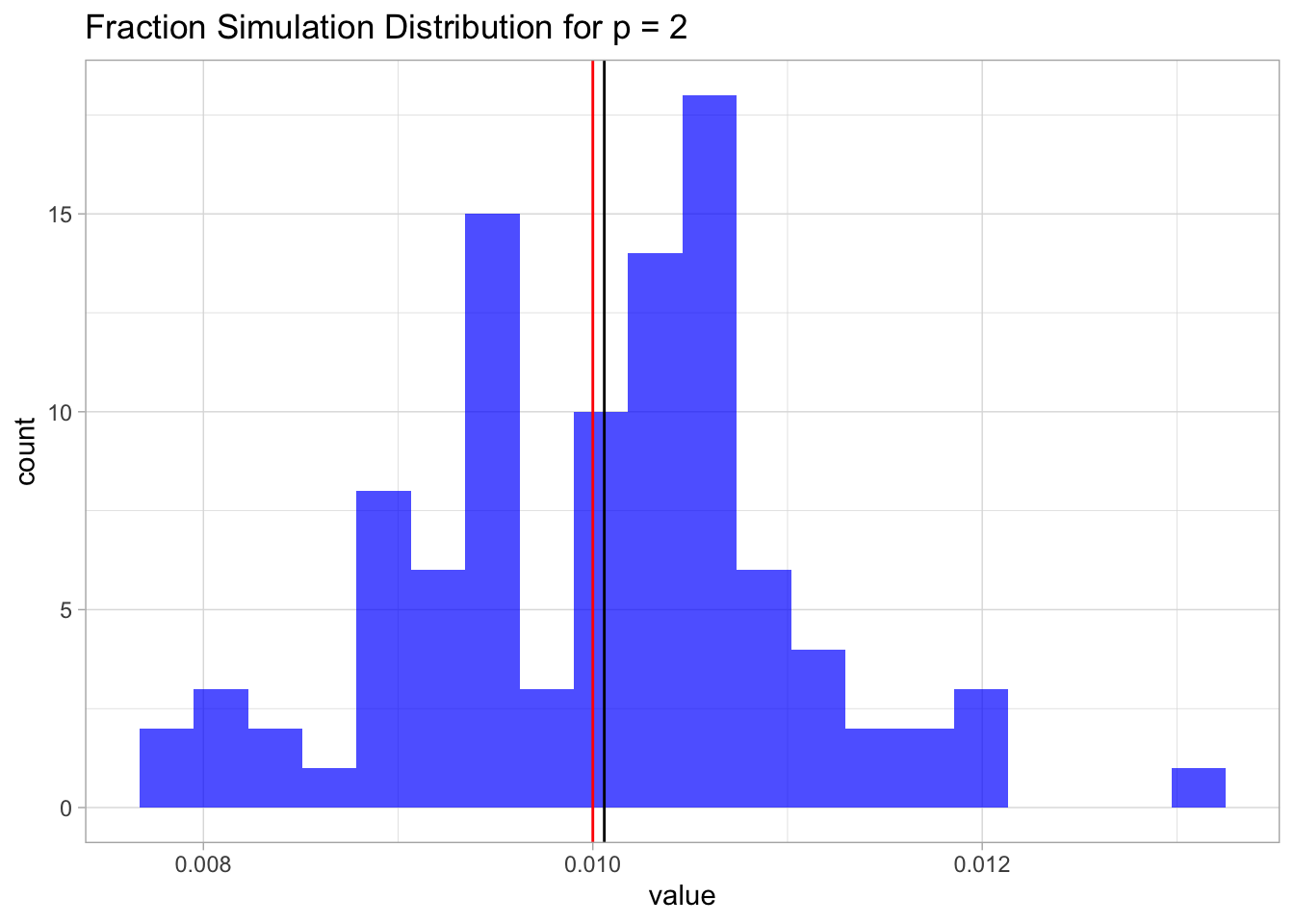1. Now suppose that we have a set of observations on $$p = 100$$ features. Again the observations are uniformly distributed on each feature, and again each feature ranges in value from $$0$$ to $$1$$. We wish to predict a test observation’s response using observations within the $$10$$% of each feature’s range that is closest to that test observation. What fraction of the available observations will we use to make the prediction?

We run the simulation first for $$p=5$$.

p <- 5

sim.p <- RunSimulationP(lambda = lambda,
p = p,
n0 = 100,
n1 = 100,
N = 100)

mean.sim.p <- mean(sim.p)

Let us plot the distribution.

sim.p %>%
as_tibble() %>%
ggplot() +
theme_light() +
geom_histogram(mapping = aes(x = value),
fill = 'blue',
alpha = 0.7,
bins = 20) +
geom_vline(xintercept = mean.sim.p) +
geom_vline(xintercept = lambda^p, color = 'red') +
ggtitle(label = glue('Fraction Simulation Distribution for p = {p}'))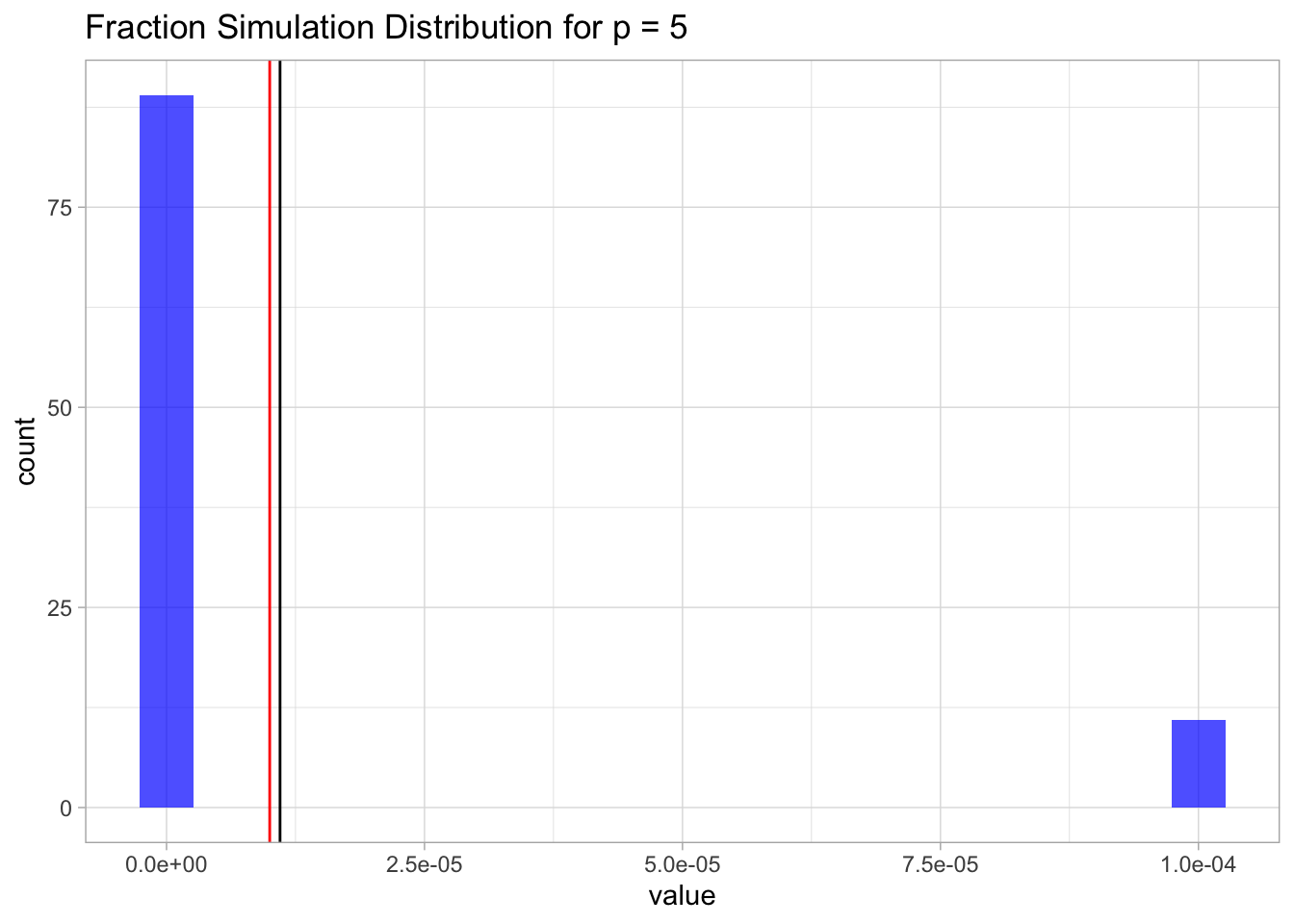For $$p=100$$ we will just get zero (a very small number).

1. Using your answers to parts (a) - (c), argue that a drawback of KNN when $$p$$ is large is that there are very few training observations “near” any given test observation.

As we have seen from (a) - (c), on average, the fraction of available observations used to make the prediction equals the volume of a $$p$$-dimensional hypercube of size $$\lambda$$, which equals $$\lambda^p$$. As this volume satisfies,

$\lim_{p\rightarrow \infty} \lambda^p = 0,$ this means that when $$p$$ is large there are very few training observations “near” any given test observation. This fact makes the prediction not robust.

1. Now suppose that we wish to make a prediction for a test observation by creating a $$p$$-dimensional hypercube centered around the test observation that contains, on average, $$10$$% of the training observations. For $$p = 1,2$$, and $$100$$, what is the length of each side of the hypercube?

In this case we want to solve for $$\lambda$$ in the equation $$\lambda^p=0.1$$, i.e. $$\lambda = 0.1^{1/p}$$.

• $$p=1$$
p <- 1

0.1^{1/p}
##  0.1
• $$p=2$$
p <- 2

0.1^{1/p}
##  0.3162278
• $$p=100$$
p <- 100

0.1^{1/p}
##  0.9772372

Let us see the values of lambda as a function of $$p$$.

tibble(Dimension = 1:100) %>%
mutate(Lambda = 0.1^(1/Dimension)) %>%
ggplot() +
theme_light() +
geom_line(mapping = aes(x = Dimension, y = Lambda)) +
geom_hline(yintercept = 1, color = 'red')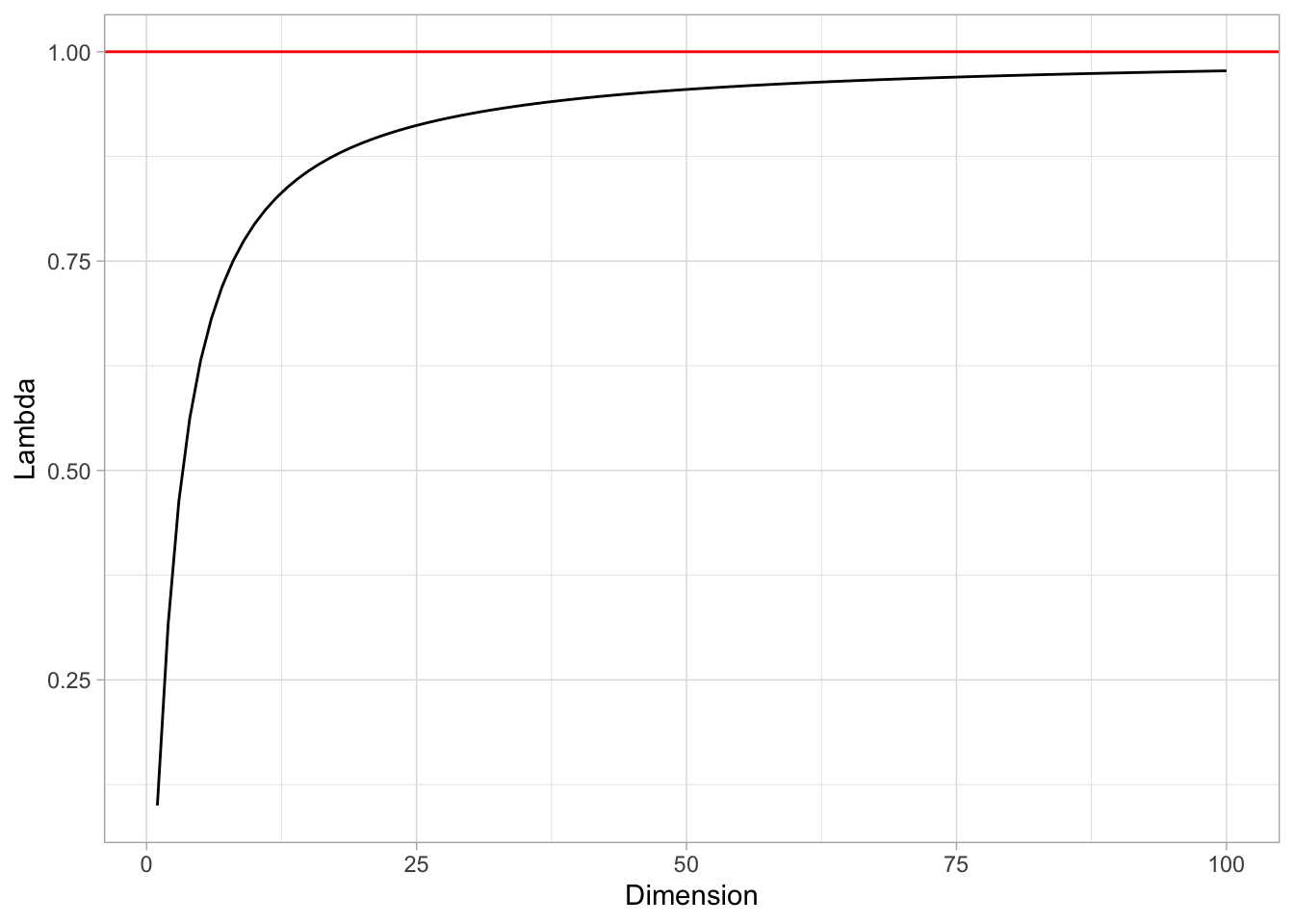That is, the side of the hypercube should grow almost to 1! This means that the “locality” is lost.

Remark: It is interesting to see that, even if the volume of the $$\lambda$$-hypercube tends to zero when $$p$$ is large, the length of its diagonal (defined as the distance between two oposite corners) grows to $$+\infty$$. Indeed, it is easy to verify that the diagonal length is $$\lambda \sqrt{p}$$ (e.g. using induction).

tibble(Dimension = 1:1000) %>%
mutate(Diagonal = sqrt(Dimension)*lambda) %>%
ggplot() +
theme_light() +
geom_line(mapping = aes(x = Dimension, y = Diagonal))